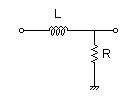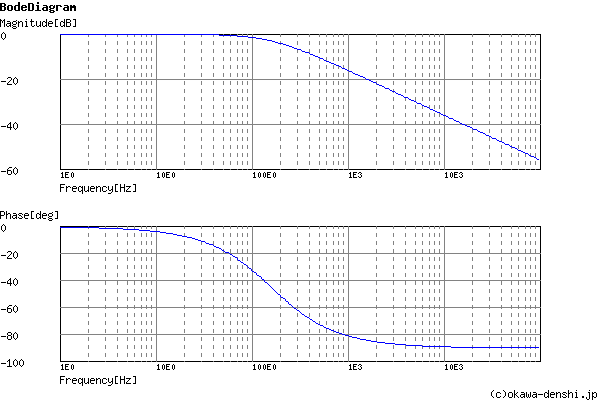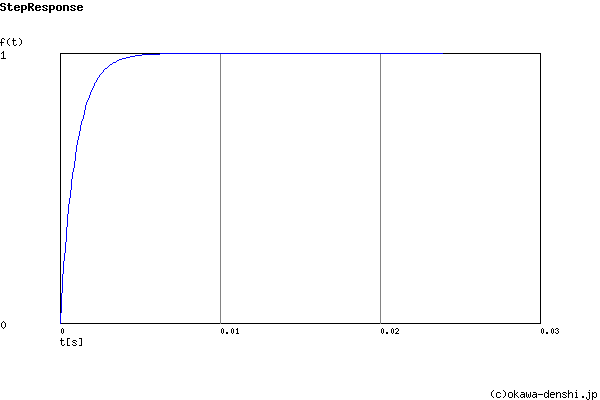# (Sample)LR Low-pass Filter Design Tool - Result -

Calculated the Transfer Function for the LR Low-pass filter, displayed on a graph, showing Bode diagram, Nyquist diagram, Impulse response and Step response.

## LR Filter

 Vin(s)→→Vout(s)
(Sample)Transfer Function:
 G(s)= 1000 s+1000

#### Cut-off frequency

fc = 159.154943092[Hz]

### Rise/Fall time of step response

R = ΩL = H

tr = [sec]

#### Pole(s)

p = -159.154943092[Hz]
|p|= 159.154943092[Hz]

#### Final value of the step response (on the condition that the system converged when t goes to infinity)

g(∞) = 1

 R=Ω L=H
p:pico, n:nano, u:micro, k:kilo, M:mega

### Frequency analysis

Bode diagram
Phase  Group delay
Nyquist diagram
Pole, zero
Phase margin
Oscillation analysis
Analysis on frequency range:
f1=∼f2=[Hz] (optional)

### Transient analysis

Step response
Impulse response
Overshoot
Final value of the step response
Analysis on time range:
0∼[sec] (optional)

# Frequency analysis# Transient analysis